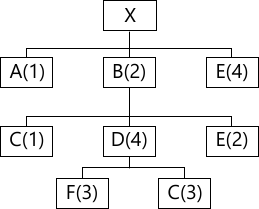MORE IN Operations Management
VTU Mechanical Engineering (Semester 8)
Operations Management
June 2015
Total marks: --
Total time: --
INSTRUCTIONS
(1) Assume appropriate data and state your reasons
(2) Marks are given to the right of every question
(3) Draw neat diagrams wherever necessary

1 (a) Explain 'Scientific Management' and its origin.
5 M
1 (b) What is Operations Management?
5 M
1 (c) What is Productivity? Mention how it can be improved.
5 M
1 (d) Suppose a company produced 300 standard book cases last week using 8 workers and it produced 240 standard book cases this week using 6 workers. In which week was productivity higher?
5 M

2 (a) Explain what 'Uncertainty' is and how this can be overcome in operations management.
8 M
2 (b) Explain 'Bayes Theorem' with notations. A study of old buses shows the probability of an accident by over speeding is 0.8, and the probability of over speeding alone is 0.3. The probability of an accident occurring due to non over speeding reduces to 0.1. Recently an accident occurred. What is the probability that the bus was overspeeding?
6 M
2 (c) Maximize 3x1+5x2, given x1+2x2≥2000, x1+x2≥1500, x2≥600 by graphical method.
6 M

3 (a) Explain the 'Moving Average' and 'Simple Exponential Smoothing' methods of forecasting.
8 M
3 (b) Explain 'Linear Regression' method for trend analysis by least squares. Explain any simplification possible.
6 M
3 (c) The data given below refers to past sales for last eleven years. Using least squares estimate sales forecast for the next two years. Also use 'Moving Average' for 3 years and compare the forecasts.
 Year 1981 1982 1983 1984 1985 1986 1987 1988 1989 1990 1991 Sales Rs. ×100 35 50 48 47 50 55 65 77 92 86 100
6 M

4 (a) How do you define 'Capacity' and how do you measure it? A factory wishes to acquire stamping machines to produce 30,000 T shirt per month. They operate 200 hours per month but the machines will be used to 75% of the time only and output is 5% defective. A stamping operation takes one minute per T-shirt. Assuming 95% efficiency, how many machines are required?
12 M
4 (b) Define 'Plant Layout'. Mention the main types of processing Layouts.
8 M

5 (a) Define 'Aggregate Planning'. Define 'Master Scheduling'. What are the differences, if any?
4 M
5 (b) Mention the strategies for 'Aggregate Planning'.
6 M
5 (c) Big Mart maintain a constant works force which can produce 3000 tables per quarter. The annual demand is 12000 units and is distributed seasonally in accordance with quarterly indexes Q1=0.80, Q2=1.40, Q3=1.00, Q4=0.80. Inventories are accumulated when demand is less than capacity and are used up during periods of strong demand. To supply the total demand i) How many tables must be accumulated each quarter? ii) What inventory must be on hand at the beginning of the first quarter?
10 M

6 (a) Define ABC analysis, EOQ, ordering cycle.
6 M
6 (b) Calculate the economic lot size with uniform rate of demand and instantaneous replacement. Give total costs and total annual costs.
6 M
6 (c) A stockiest has to supply 800 units per week of a product to his customers. He gets the product at RS.75 per unit from the manufacturer. The cost of ordering and transportation from the manufacturer is Rs. 100 per order. The cost of carrying is 10% per year of the cost of the product. Calculate the economic lot size, time to produce the economic lot size and total optimum cost per week.
8 M

7 (a) What is MRP and give the inputs required?
4 M
7 (b) What is ERP?
4 M
7 (c) A work centre operates 6 days a week on a two shifts per day basis (8 hours per shift). It has four machines with the same capacity. If the machines are utilized 75% of the time at a system efficiency of 90%, what is the rated output in standard hours per week?
6 M
7 (d) Give the structure tree shown, compute the net requirements of A, B, C, D, E, F to produce 10 units of end item X. No stock is on hand.6 M

8 (a) Explain the importance of purchase and supply management.
6 M
8 (b) Explain the concept of tenders and logistics management.
6 M
8 (c) Micro-Brush requires a new component for their laptop cleaning machines. The company must decide whether to make or buy them. If it decides to make them. Should it use process A or process B? Use a Break-Even analysis to advise them if the following data is given:
i) Should Micro make using process A or B or buy?
ii) At what annual volumes should Micro switch from each make/buy decision to the other?
 Make A Make B Buy Annual volume 10,000 10,000 10,00 Fixed cost/year Rs. 150,000 Rs. 2,00,000 Variable cost/unit Rs. 100 Rs. 50 Rs. 200
8 M

More question papers from Operations Management